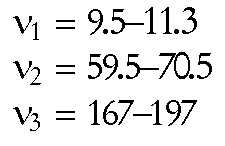Solutions to Homework Assignment #1

1. (Real) cube root by Newton's iteration

2. Complex arithmetic functions (in Basic for a change)

3. Laguerre algorithm in Forth

4. Solution to problem in *.ps formatEigenfrequencies of vibrating rod (Hz)